Function Repository Resource:

# LemoineInellipse

Generate the Lemoine inellipse of a 2D triangle

Contributed by: Jan Mangaldan
 ResourceFunction["LemoineInellipse"][{p1,p2,p3}] returns an Ellipsoid representing the Lemoine inellipse of the triangle defined by vertices p1,p2 and p3. ResourceFunction["LemoineInellipse"][{p1,p2,p3},property] gives the value of the specified property.

## Details

The Lemoine inellipse of a triangle is the unique ellipse inscribed in a triangle whose foci are the triangle's centroid and Lemoine point.
The following properties are supported:
 "Ellipsoid" Ellipsoid representing the inellipse "Parametric" parametric equation for the inellipse as a pure function "Implicit" implicit Cartesian equation for the inellipse as a pure function
ResourceFunction["LemoineInellipse"][poly] where poly is a Triangle or Polygon is equivalent to ResourceFunction["LemoineInellipse"][PolygonCoordinates[poly]].

## Examples

### Basic Examples (1)

Show a triangle together with its Lemoine inellipse:

 In:=Out=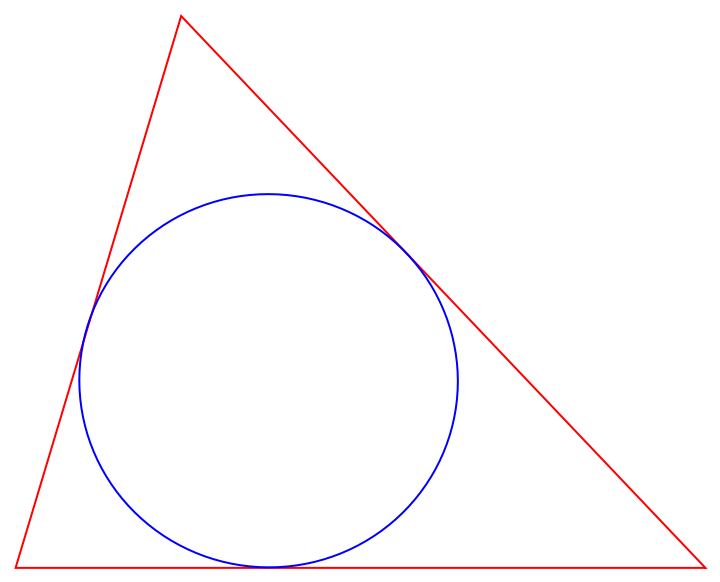### Scope (5)

A triangle:

 In:=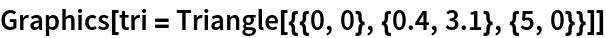Out=Generate the parametric equation of the triangle's Lemoine inellipse:

 In:=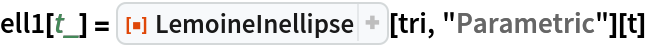Out=Plot the parametric equation along with the triangle:

 In:=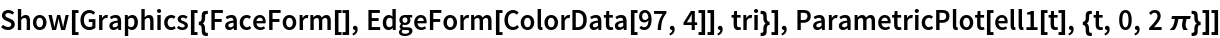Out=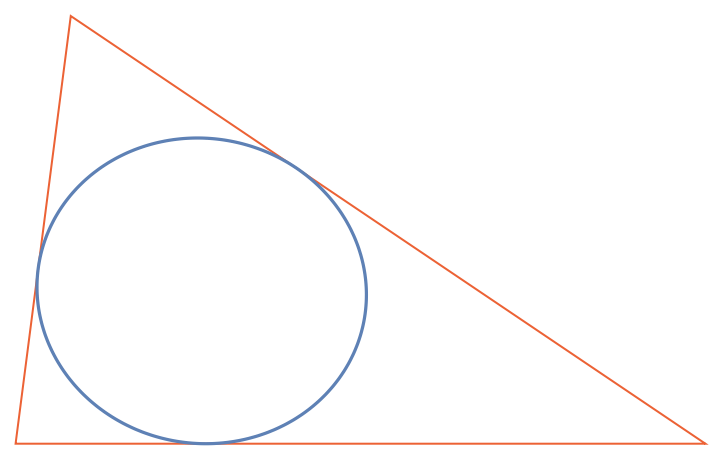Generate the implicit equation of the triangle's Lemoine inellipse:

 In:=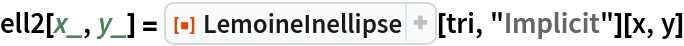Out=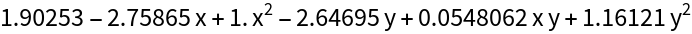Plot the implicit equation along with the triangle:

 In:=Out=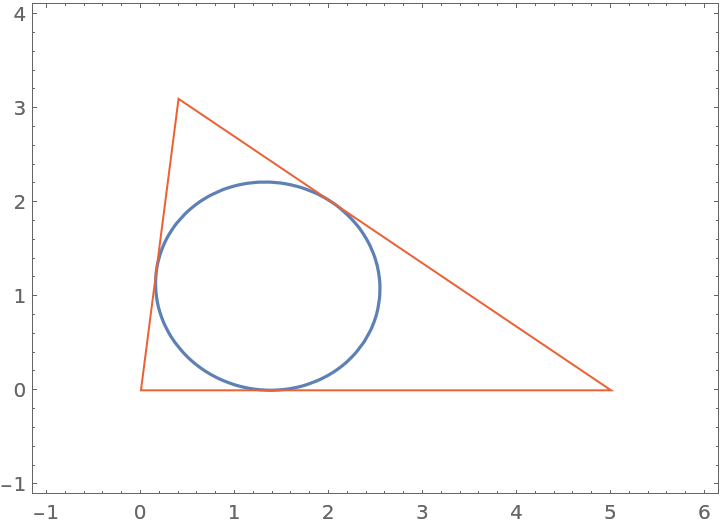### Properties and Relations (2)

Use the resource function EllipseProperties to generate properties of the inellipse:

 In:=Out=Give the foci of the inellipse:

 In:=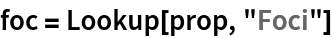Out=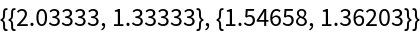One focus corresponds to the centroid:

 In:=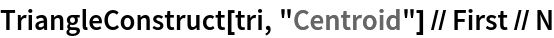Out=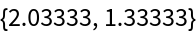The other focus corresponds to the symmedian point (Lemoine point):

 In:=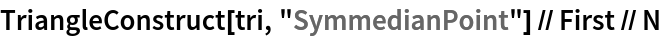Out=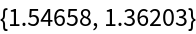The area of the Lemoine inellipse can be expressed in terms of the area of the original triangle and the lengths of its sides:

 In:=Out=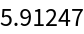In:=Out=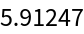## Version History

• 1.0.0 – 13 September 2021